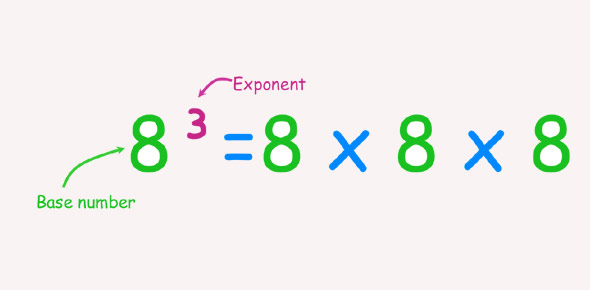# Chapter 8 Exponents Quiz

10 Questions | Total Attempts: 232Settings• 1.
52    + 3  =
• 2.
33    --   7 =
• 3.
2  +   42 =
• 4.
24   + 5 =
• 5.
62  +  4 =
• 6.
103 --  400 =
• 7.
72  + 1 =
• 8.
Which of the following numbers is prime?
• A.

14

• B.

51

• C.

47

• D.

27

• 9.
Which of the following numbers is composite?
• A.

2

• B.

19

• C.

17

• D.

15

• 10.
Which of the following numbers is neither prime nor composite?
• A.

7

• B.

1

• C.

9

• D.

11

Related TopicsBack to top